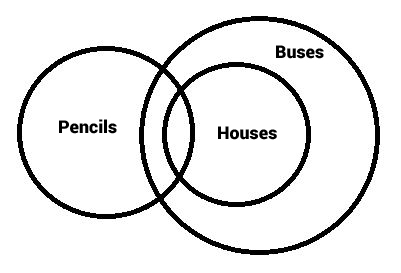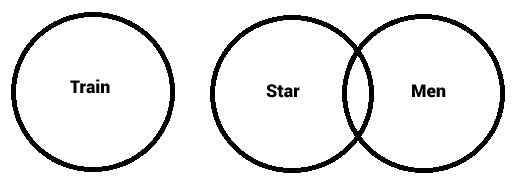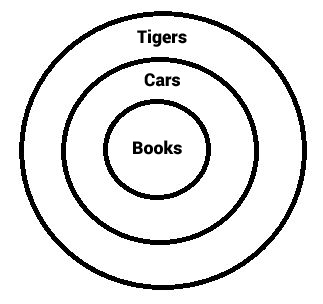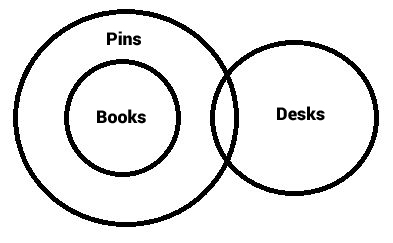# Syllogism Practice Questions for IBPS Clerk (Set-2)

0
3800

Syllogism Practice Questions for IBPS Clerk (Set-2):

Instructions:

In each of the questions below are given two statements followed by three conclusions numbered I, II and III. You have to take the given statements to be true even if they seem to be variance from commonly-known facts. Read all the conclusions and then decide which of the given conclusions logically follows from the given statements disregarding commonly-known facts.

Question 1:

Statements:
Some pencils are houses
All houses are buses.
Conclusions:
I. Some pencils are buses.
II. Some buses are pencils.
III. All buses are pencils

a) All follow
b) None follows
c) Only II follows
d) Only I and II follow
e) None of these

Question 2:

Statements:
No train is star
Some stars are men.
Conclusions:
I. Some men are trains
II. No men is train
III. All men are stars

a) None follows
b) Only I follows
c) Only either I or II follows
d) Only either II or III follows
e) None of these

Question 3:

Statements:
Conclusions:
II. Some stones are trees
II. No stone is tree

a) Only either II or III follows
b) Only either I or II follows
c) Only either I or III follows
d) Only II follows
e) None of these

Question 4:

Statements:
All books are cars
All cars are tigers
Conclusions:
I. All books are tigers
II. All tigers are books
III. All cars are books

a) Only I follows
b) Only II follows
c) Only I and II follow
d) All follow
e) None of these

Question 5:

Statements:
All books are pins
Some pins are desks
Conclusions:
I. Some desks are books
II. All desks are pins
III. No desk is book

a) Only either I or II follows
b) Only either I or III follows
c) Both I and III follow
d) Only I follows
e) None of these

Solutions (1 to 5)We use elimination to find an exception to the generality of the conclusions. Thus we prove they are not implied. The diagram above satisfies all the statements but contradicts conclusion III. Since we found an exception, the conclusion is not true in “every” case. Thus it is not implied.
We can draw many scenarios that satisfy the statements using Venn diagrams and check for the validity of the conclusions. Conclusions I and II hold in every case. So, they are implied.We use elimination to find an exception to the generality of the conclusions. Thus we prove they are not implied. The diagram above satisfies all the statements but contradicts conclusion III. Since we found an exception, the conclusion is not true in “every” case. Thus it is not implied.
Irrespective of the diagram, conclusions I and II are universally complimentary ie. one of the two always has to be implied but both can’t be true at the same time. Hence we use “either” and “or”.

We use elimination to find an exception to the generality of the conclusions. Thus we prove they are not implied. The diagram below satisfies all the statements but contradicts conclusions I and III. Since we found an exception, the conclusions are not true in “every” case. Thus they are not implied.
We can draw many scenarios that satisfy the statements using Venn diagrams and check for the validity of the conclusions. Conclusion II holds in every case. So, it is implied.